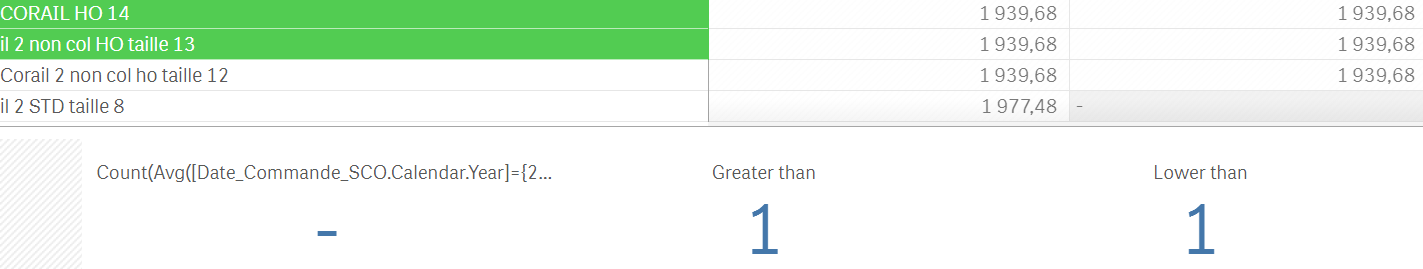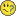New to Qlik Sense

Discussion board where members can get started with Qlik Sense.

Announcements
QlikWorld 2020: Join us May 11 - 14, 2020 in Phoenix, AZ. Register early and save \$400. Learn MoreNot applicable

Price variation - Count function

Hi everyone,

I do use a Count funciton in a KPI object to calculate the number of prices which went up over the laster year but I cannot make it with my current formula!

Count(Avg([Year]={2016})Price >Avg([Year]={2017})Price)

Do you have any tips or advices?

Thanks a lot

Florian

1 Solution

Accepted SolutionsMVP

Re: Price variation - Count function

What is the expression you are using? May be you need to use Rounding here? Round to 2 digits for matching?

18 RepliesMVP

Re: Price variation - Count function

May be this

Sum(Aggr(If(Avg({<[Year]={2016}>}Price) > Avg({<[Year]={2017}>}Price), 1, 0), ProductID))

Assuming we are talking about the prices for a product. Change this to a field for which you are doing the price comparisonMVP

Re: Price variation - Count function

For prices that went up... you might need this

Sum(Aggr(If(Avg({<[Year]={2016}>}Price) < Avg({<[Year]={2017}>}Price), 1, 0), ProductID))Not applicable

Re: Price variation - Count function

Hi Sunny,

Thank you for your answer and your reactivityI do get a figure in my KPI but which does not work exactly. I do have a lot of mising data for 2017 and my KPI takes some of them into account. Shall I include an IF within my current function to sort this out?

Thank you again

Cheers

FlorianNot applicable

Re: Price variation - Count function

Look at the two selected fields, one of them gives me the result "greater than" and the second "lower than" and I need them to be ricorded as equal.

It might be an very small difference or simply the function which doesn't work as hopedBest,

FlorianMVP

Re: Price variation - Count function

I am not completely sure I understand what you are trying to convey here. Can you elaborate?Not applicable

Re: Price variation - Count function

Hi Sunny,

So you might be confused with the names "Greater than" & "Lower than". My PivotTable aims to reflect the number of prices which are going up (greater than) and down (lower than)  between 2016 (1st column) and 2017 (2nd column).

I am trying to find the correct formula to display this result within my two KPI boxes.

Do you have an idea how I could edit my formula to get the correct answer?

Thanks,

FlorianMVP

Re: Price variation - Count function

What is the expression you are using? May be you need to use Rounding here? Round to 2 digits for matching?Not applicable

Re: Price variation - Count function

Yeah thank you, it seems to work for these references but my formulation takes sometime the null value into account(as zero value I guess).

If you have a look at the following table you will see one single selected field which should not increase my Delflation KPI. Do you have an idea how to solve this? Using a null() function might be appropriate in this case, right?My KPI formula:

Sum(Aggr(If(round(Avg({<[Year]={2016}>}Price),0.01) > round(Avg

({<[Year]={2017}>}Price),0.01), 1, 0),Supplier))

I tried to insert a null() function but it doesn't really work:

Sum(Aggr(If(if(null(Avg({<[Year]={2016}>}Price)),0, round

(Avg({<[Year]={2016}>}Price),0.01)) < if(null

(Avg({<[Year]={2017}>}Price)),0, round(Avg

({<[Year]={2017}>}Price),0.01)), 1, 0),Supplier))

Moreover, I am using this expression for the color format. If you look at my table the Delta (-0.46%) is not displayed in red as I wish, is the round() function not used correctly?

if((Avg({1<[Year]={2017} >}Price)-Avg({1<[Year]

={2016} >}Price))/Avg({1<[Year]={2016} >}Price)>0,black(),if((Avg({1<[Year]={2017} >}Price)-Avg({1<[Year]

={2016} >}Price))/Avg({1<[Year]={2016} >}Price)<0,black(),))

It would be wonderful if you had an idea how I could get out this messThanks a lotFlorianMVP

Re: Price variation - Count function

May be try this

Sum(Aggr(If(Round(Avg({<[Year] = {2016}>} Price), 0.01) > Round(Avg({<[Year] = {2017}>} Price), 0.01) and Len(Trim(Avg({<[Year]={2016}>}Price))) > 0 and Len(Trim(Avg({<[Year]={2016}>}Price))) > 0, 1, 0), Supplier))

Where are you specifying the red color in this expression? All I see is black()?

if((Avg({1<[Year]={2017} >}Price)-Avg({1<[Year]

={2016} >}Price))/Avg({1<[Year]={2016} >}Price)>0,black(),if((Avg({1<[Year]={2017} >}Price)-Avg({1<[Year]

={2016} >}Price))/Avg({1<[Year]={2016} >}Price)<0,black(),))